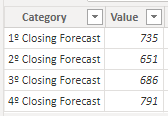cancel
Showing results for
Did you mean:Helper III

## Growth percentage by category

Hi guys.

How could I get the following result? For example, I would like to calculate the difference and growth in percentage of the 1st Closing Forecast in relation to the 2nd Closing Forecast, the 2nd Closing Forecast in relation to the 3rd Closing Forecast, but I am not using any calendar table, only table category.

Example:
1º Closing Forecast (735)
2º Closing Forecast (651)
Difference = 84

growth in percentage = - 12%1 ACCEPTED SOLUTIONCommunity Support

Hi @kasife ,

According to your description, here are my steps you can follow as a solution.

(1) This is my test data.(2)Go into the power query editor and copy and paste the code into the "Advanced Editor".

``````let
Source = Table.FromRows(Json.Document(Binary.Decompress(Binary.FromText("i45WMjy0S8E5J784My9dwS2/KDU5sbhESUfJ3NhUKVYnWskIu7SZqSFY2hiHtIUZWNoEh+GWQN2xAA==", BinaryEncoding.Base64), Compression.Deflate)), let _t = ((type nullable text) meta [Serialized.Text = true]) in type table [Category = _t, Value = _t]),
#"Changed Type" = Table.TransformColumnTypes(Source,{{"Category", type text}, {"Value", Int64.Type}}),
#"Duplicated Column" = Table.DuplicateColumn(#"Changed Type", "Category", "Category - Copy"),
#"Split Column by Delimiter" = Table.SplitColumn(#"Duplicated Column", "Category - Copy", Splitter.SplitTextByDelimiter("º Closing Forecast", QuoteStyle.Csv), {"Category - Copy.1", "Category - Copy.2"}),
#"Changed Type1" = Table.TransformColumnTypes(#"Split Column by Delimiter",{{"Category - Copy.1", Int64.Type}, {"Category - Copy.2", type text}}),
#"Removed Columns" = Table.RemoveColumns(#"Changed Type1",{"Category - Copy.2"}),
#"Renamed Columns" = Table.RenameColumns(#"Removed Columns",{{"Category - Copy.1", "Category - Index"}})
in
#"Renamed Columns"``````

(3) We can create a calculated column.

``````Column =
IF (
LOOKUPVALUE ( 'Table'[Value], 'Table'[Category - Index], 'Table'[Category - Index] - 1 )
= BLANK (),
0,
(
'Table'[Value]
- LOOKUPVALUE ('Table'[Value], 'Table'[Category - Index], 'Table'[Category - Index] - 1)
)
/ LOOKUPVALUE ( 'Table'[Value], 'Table'[Category - Index], 'Table'[Category - Index] - 1 )
)``````

(4) Then the result is as follows.If the above one can't help you get the desired result, please provide some sample data in your tables (exclude sensitive data) with Text format and your expected result with backend logic and special examples. It is better if you can share a simplified pbix file. Thank you.

Best Regards,

Neeko Tang

If this post  helps, then please consider Accept it as the solution  to help the other members find it more quickly.

2 REPLIES 2Community Support

Hi @kasife ,

According to your description, here are my steps you can follow as a solution.

(1) This is my test data.(2)Go into the power query editor and copy and paste the code into the "Advanced Editor".

``````let
Source = Table.FromRows(Json.Document(Binary.Decompress(Binary.FromText("i45WMjy0S8E5J784My9dwS2/KDU5sbhESUfJ3NhUKVYnWskIu7SZqSFY2hiHtIUZWNoEh+GWQN2xAA==", BinaryEncoding.Base64), Compression.Deflate)), let _t = ((type nullable text) meta [Serialized.Text = true]) in type table [Category = _t, Value = _t]),
#"Changed Type" = Table.TransformColumnTypes(Source,{{"Category", type text}, {"Value", Int64.Type}}),
#"Duplicated Column" = Table.DuplicateColumn(#"Changed Type", "Category", "Category - Copy"),
#"Split Column by Delimiter" = Table.SplitColumn(#"Duplicated Column", "Category - Copy", Splitter.SplitTextByDelimiter("º Closing Forecast", QuoteStyle.Csv), {"Category - Copy.1", "Category - Copy.2"}),
#"Changed Type1" = Table.TransformColumnTypes(#"Split Column by Delimiter",{{"Category - Copy.1", Int64.Type}, {"Category - Copy.2", type text}}),
#"Removed Columns" = Table.RemoveColumns(#"Changed Type1",{"Category - Copy.2"}),
#"Renamed Columns" = Table.RenameColumns(#"Removed Columns",{{"Category - Copy.1", "Category - Index"}})
in
#"Renamed Columns"``````

(3) We can create a calculated column.

``````Column =
IF (
LOOKUPVALUE ( 'Table'[Value], 'Table'[Category - Index], 'Table'[Category - Index] - 1 )
= BLANK (),
0,
(
'Table'[Value]
- LOOKUPVALUE ('Table'[Value], 'Table'[Category - Index], 'Table'[Category - Index] - 1)
)
/ LOOKUPVALUE ( 'Table'[Value], 'Table'[Category - Index], 'Table'[Category - Index] - 1 )
)``````

(4) Then the result is as follows.If the above one can't help you get the desired result, please provide some sample data in your tables (exclude sensitive data) with Text format and your expected result with backend logic and special examples. It is better if you can share a simplified pbix file. Thank you.

Best Regards,

Neeko Tang

If this post  helps, then please consider Accept it as the solution  to help the other members find it more quickly.Helper III

Its working. Thanks @v-tangjie-msft